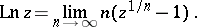# Logarithmic function

logarithm

The function inverse to the exponential function. The logarithmic function is denoted by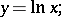(1)

its value, corresponding to the value of the argument, is called the natural logarithm of. From the definition, relation (1) is equivalent to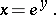(2)

Since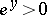for any real, the logarithmic function is defined only for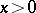. In a more general sense a logarithmic function is a functionwhere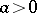(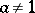) is an arbitrary base of the logarithm; this function can be expressed in terms of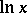by the formula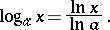The logarithmic function is one of the main elementary functions; its graph (see Fig.) is called a logarithmic curve.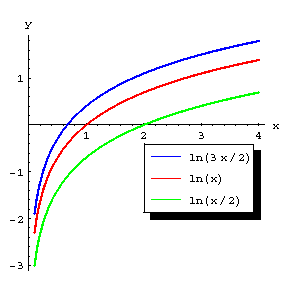Figure: l060600a

The main properties of the logarithmic function follow from the corresponding properties of the exponential function and logarithms; for example, the logarithmic function satisfies the functional equation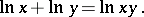The logarithmic functionis a strictly-increasing function, and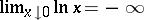,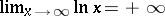. At every pointthe logarithmic function has derivatives of all orders and in a sufficiently small neighbourhood it can be expanded in a power series, that is, it is an analytic function. For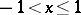the following expansion of the (natural) logarithmic function is valid: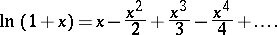The derivative of the logarithmic function is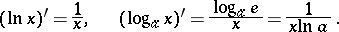Many integrals can be expressed in terms of the logarithmic function; for example: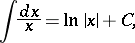The dependence between variable quantities expressed by the logarithmic function was first considered by J. Napier in 1614.

The logarithmic function on the complex plane is an infinitely-valued function, defined for all values of the argument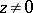, and is denoted by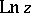(or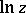if no confusion arises). The single-valued branch of this function defined by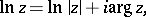whereis the principal value of the argument of the complex number,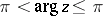, is called the principal value of the logarithmic function. One has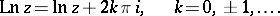All values of the logarithmic function for negative realare purely imaginary complex numbers. The first satisfactory theory of the logarithmic function for complex arguments was given by L. Euler in 1749; he started from the definition